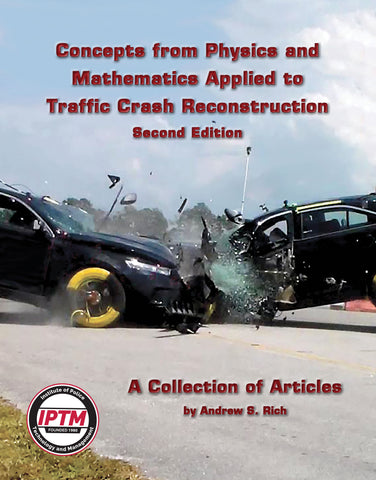# Concepts from Physics and Mathematics Applied to Traffic Crash Reconstruction Second Edition

• \$ 25.00

### Concepts from Physics and Mathematics Applied to Traffic Crash Reconstruction - Second Editionby Andrew S. Rich

This collection of articles delves into various areas of crash reconstruction. The first article provides you with a solid foundation for solving systems of equations which you can apply to problems involving conservation of linear momentum, conservation of energy and collinear momentum problems.

As the publication dates of the articles progress, the topics become more complex. You will find articles discussing a method to solve the airborne equation when the takeoff angle is not known; a special case in the conservation of collinear momentum; equivalent barrier speed; equivalent energy speed and delta-V; and a geometric analysis for estimating collision force for narrow-object impacts. In an expanded section on the Monte Carlo method, Mr. Rich provides step-by-step instructions for completing Monte Carlo analyses in Microsoft® Excel®, as well as sample ROOT scripts for a couple of scenarios that perform simple Monte Carlo analyses complete with histograms of the results.

Chapters include:

• Solving Second-Degree and Systems of Equations: Applications to Motor Vehicle Accident Reconstruction (1998)
• A Three-Point Airborne Trajectory Analysis: An Application of the Derivative (1999)
• A Special Case in the Conservation of Collinear Momentum (2003)
• Momentum Revisited (2003)
• An Introduction to the Monte Carlo Method (2005-2020)
• EBS and Delta V (2006)
• A Geometric Analysis for Estimating Collision Force for Narrow Object Impacts (2020 Updated)